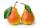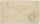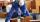# Garden

Father dig up the garden in 9 hours. Son in 13 hours. How many hours take dig up the garden together?

Correct result:

x =  5.3182 h

#### Solution:

x/9+x/13 = 1

22x = 117

x = 11722 ≈ 5.318182

Our simple equation calculator calculates it.We would be pleased if you find an error in the word problem, spelling mistakes, or inaccuracies and send it to us. Thank you!Tips to related online calculators
Looking for calculator of harmonic mean?
Looking for a statistical calculator?
Need help calculate sum, simplify or multiply fractions? Try our fraction calculator.
Do you have a linear equation or system of equations and looking for its solution? Or do you have quadratic equation?
Do you want to convert time units like minutes to seconds?

## Next similar math problems:

• Simple equationSolve for x: 3(x + 2) = x - 18
• Cupcakes 2Susi has 25 cupcakes. She gives 4/5. How much does she have left?
• Simple equation 9Solve the following equation: -8y+5=-9y+9
• OrchardThree-fifths of the orchard are apple trees, one-third of the trees are cherries. The remaining 5 trees are pears. How many trees are in the orchard?
• AuntAnnie aunt is 35 years old, Annie is 14 years. For how many years will aunt have twice as many years as Annie?
• Grandmother and grandfatherGrandmother baked cakes. Grandfather ate half, then quarter of the rest ate Peter and Paul ate half of rest. For parents left 6 cakes. How many cakes maked the grandmother?
• FractionsThree-quarters of an unknown number are 4/5. What is 5/6 of this unknown number?
• Equation 20In given equation: 8/9-4/5=2/9+x, find x
• Cleaning windowsCleaning company has to wash all the windows of the school. The first day washes one-sixth of the windows of the school, the next day three more windows than the first day and the remaining 18 windows washes on the third day. Calculate how many windows ha
• Simple equation 6Solve equation with one variable: X/2+X/3+X/4=X+4
• Bricklayers8 bricklayers build a house for 630 days. How many bricklayers have to add after 150 days, so then the whole building completed in (next) 320 days?
• Two machinesThe first machine can produce parts in 24 hours, the second in 16 hours. The first started working in the morning at 6:00 pm. Second at 10:00 o'clock. At what hour were all parts made?
• Fraction + eqSolve following simple equation with fractions: -5/6(8+5b) = 75 + 5/3b
• Greg and BillGreg is 18 years old. He is 6 less than 4 times Bill's age. How old is Bill?
• NovakNovak needed to dig up three of the same pit in the garden. The first pit dug father alone for 15 hours. His second dig son helped him and it did that in six hours. The third pit dug son himself. How long it took him?
• Simple equation 324 = n • 27, solve for n
• SoldierAn experienced soldier digs a good trench in 30 hours. Two soldiers dug the same trench each 40 hours. How many hours will the ditch dig all three together?